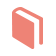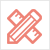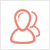﻿ Essay范文-American options are financial derivatives-51Due留学教育

#### 服务承诺资金托管原创保证实力保障24小时客服使命必达

#### 关于我们

51Due提供Essay，Paper，Report，Assignment等学科作业的代写与辅导，同时涵盖Personal Statement，转学申请等留学文书代写。51Due将让你达成学业目标51Due将让你达成学业目标51Due将让你达成学业目标51Due将让你达成学业目标

#### 名企实习积累工作经验多元化文化交流专业实操技能建立人际资源圈

# American options are financial derivatives

2020-12-30 来源: 51Due教员组 类别: Essay范文

American Options Are Finanical Derivatives

American options are financial derivatives, an instrument whose value is derived from an underlying asset, usually a stock. Black and Scholes (1973) described an option as: "a security giving the right to buy or sell an asset, subject to certain conditions, within a specified period of time".

The main question of this dissertation is how American options can be valued. The option value is only known with certainty when the option is exercised, either at maturity or not. When the owner decides to exercise the option or it is the option maturity time, it is possible to determine the price of the option as the strike will be exchanged by the asset in the case that the conditions are favourable for the owner of the option. When the one buys the option, she does not know what will be the future price of the underlying asset, and assuming it follows a random process it is hard to put a price on such contract without knowing what will be the price change. This non linear feature of the option makes calculating the price to pay for such contracts a challenging process and has been the focus of a large number of financial studies and publications.

This dissertation deals with the most popular methods for pricing American options and their implementation in MatLab?, including a graphic user interface.

The methods studied include the Black and Scholes (1973) European option pricing as the starting point, followed by the Barone Adesi and Whaley (1987) analytical approximation. Then the binomial and trinomial lattice methods presented in Cox, Ross and Rubinstein (1979) are considered also as the Finite difference approximations models AAA. The most sophisticated method is the Least Squares Monte Carlo simulation presented in Longstaff and Schwartz (2001).

The analysis of the different option pricing methods in this dissertation follow most of the assumptions made by Black and Scholes (1973), the short term interest rate and the dividend are assumed to be known and constant, the underlying stock follows a log normal distributed geometric Brownian motion, the markets are frictionless and finally it exists the possibility of forming a riskless portfolio, consisting of the option and underlying stock.

The dissertation is organised as follows: a brief literature survey is provided in the next Chapter. The analytical approximation method and the numerical methods used are described on Chapter 3 and their implementation in Matlab environment is given in chapter 4. Numerical results are given in Chapter 5. The conclusion and future developments are presented in Chapter 6.

Chapter 2 provides a survey of some of the most relevant publications in American Option Pricing, with focus on analytical approximations, lattice and finite difference methods, more precisely, binomial and trinomial trees, explicit, implicit and Crank Nicolson Scheme, and also on Monte Carlo Simulation.

Chapter 3 provides a description of the methods used, their advantages, disadvantages and limitations. Here the required equations will be derived and the solution for the pricing of American options will be provided.

Chapter 4 focus on the algorithms used and their implementation on the MatLab environment, also as the procedures for the development of the GUI for easier user interface.

On Chapter 5 results and their comparison are shown for the different methods used, with the required figures to support the numerical answers.

In the final chapter the dissertation is concluded and a summary of the findings is provided, also as with further work on this subject.

Black and Scholes (1973) and Merton (1973) developed the first analytical closed form solution for the pricing of European type options and certain types of American options, such as American call options on non dividend paying stocks. "The option pricing model developed by Black and Scholes and extended by Merton gives rise to partial differential equations governing the value of an option" Schwartz (1976).

Black and Scholes (1973) develop their model on the basis of the no arbitrage theory, "If options are correctly priced in the market, it should not be possible to make sure profits by creating portfolios of long and short positions in options and their underlying stocks" Black and Scholes (1973).

The Black and Scholes (1973) model valued European options on non dividend paying stocks, and with a number of quite restrictive assumptions, constant and known interest rates, the markets are frictionless with no transaction costs and penalties for short selling. The Black and Scholes (1973) model also assumes that the underlying stocks follow a random walk. Due to all this assumptions the pricing model Black and Scholes (1973) proposed was of easy use, and there is only the need to input the required values on the proposed pricing equation. The model they have proposed does not take into consideration early exercise of the option so it is inaccurate for pricing American Options.

One of the most popular analytical approximation models that starts from the Black and Scholes (1973) model and adjusts it to consider the scenario of early exercise strategies is the work by Baron Adesi and Whaley (1987) which was based on the paper by MacMillan (1986).

Baron Adesi and Whaley (1987) consider that the Black and Scholes (1973) partial differential equation must apply to the early exercise premium as this is just the difference between the American and the European option prices, which are also priced by the same partial differential equation. After some transformation they end with an easily solvable through an interactive process second order differential equation.

When closed form solutions, like the Black and Scholes (1973) valuation model cannot be derived, numerical methods must be developed. These are computational methods where the values for the underlying assets are modelled up to maturity and the price of the options is derived from them. In the case of American options this is a complex process, as the modelled price changes may have to be adjusted to include dividend payments and the derivation of the option price must also include the possibility of early exercise.

Cox, Ross and Rubinstein (1979) developed a simple discrete time lattice model to deal with the complexity of option valuation, as they considered the methods of Black and Scholes (1973) "quite advanced and have tended to obscure the underlying economics" Cos, Ross and Rubinstein (1979). The use of lattice models such as the one by Cox, Ross and Rubinstein (1979) is the simplicity of its application.

The most significant drawback of the Cox, Ross and Rubinstein (1979) model, is to increase its accuracy the number of time intervals must increase, in order to approach a continuous time model, which will significantly increase the computational time, needed for processing the entire tree in order to derive the option value.

Others such as Hull and White (1988), (1993) and Trigeorgis (1991) have extended the model of Cox, Ross and Rubinstein (1979).

Hull and White (1988) present a study of the use of lattice models for underlying assets with known dividends instead of known divided yields. They also consider the use of a control variate to price a option numerically, by a the lattice model, using the price of a similar option calculated analytically. While Trigeorgis (1991) proposes "a log transformed variation of binomial option pricing designed to overcome problems of consistency, stability and efficiency encountered in the Cox, Ross and Rubinstein (1979)" focusing on the pricing of exotic options. Hull and White (1993) also present an application of binomial and trinomial procedures for exotic path dependent options, where they developed a model faster than Monte Carlo simulation and faster than other numerical methods.

Usually the analytical procedures are applicable to simple payoffs of the American Options, but in the cases where this is not possible numerical solutions must be developed. Geske and Shastri (1985) give a detailed comparison of the lattice methods to the different numerical methods, finite difference methods and other simulation methods.

The model proposed by Brennan and Schwartz (1978) for valuing options was the first approach that used the finite difference method. This approach was used due to the fact that most of the times an analytical solution for the option pricing problem does not exist. The finite difference method uses the heat equation derived from the Black and Sholes PDE to obtain an approximation of the option price. Courtadon (1998) goes further to reduce the approximation error of the Brennan and Schwartz (1978) model but only applies his findings only to simple option pay offs.

Geske and Shastri (1985) give a good description of the finite difference method: "The finite difference technique analyze the partial differential equation (...) by using discrete estimates of the changes in the options value for small changes in time or the underlying stock price to form equations as approximations to the continuous partial derivatives." Usually the approximations is done using forward, backward or central difference theorem, which respectively result in the explicit, implicit and Crank Nicolson schemes, the procedure used in this study will be shown further in the paper.

In this case as with most of the methods for pricing options, the most significant drawback is the duality between accuracy and processing time. In order to increase accuracy the time and stock change steps must be smaller, increasing their number and the number of computations to make, this issue also affects the stability and convergence of the methods.

Another approach used for solving the option pricing problem, especially for path dependent American options is the use of simulation. This means that the option price is derived from a simulated underlying asset price, usually using a Monte Carlo simulation method. Boyle (1977) and Schwartz (1977) pioneered the use of Monte Carlo simulation which is nowadays used to price complex options contracts. The Monte Carlo simulation method is very powerful in terms of its flexibility to generate the returns of the underlying asset of the options, by changing the random variables used to generate the process a new returns distribution may be easily obtained, Boyle (1977).

Boyle (1977) introduces the Monte Carlo technique for pricing European option where there is a dividend payment, but Schwartz (1977) was the true pioneer, pricing American options, with the underlying asset paying discrete dividends, and also deriving an optimal strategy for early exercise of the option, which is the crucial point for pricing American type options. Schwartz (1997) focused on a particular type of contract, warrants, so in fairness his first model is not exactly on an American type option.

Tilley (1993) was one of the first to fully focus on the pricing of American option using a Monte Carlo simulation method as he mentioned that simulation methods were reserved for exotic options or other complex debt products. His findings are only applied to American options on non dividend paying stocks, but he develops an important part of the model which is the optimal early exercise option.

Carriere (1996) presents a development of the Monte Carlo simulation method presented by Tilley (1993). The paper by Carriere (1996) presents a model where the optima early exercise strategy is based on conditional expectations of Markov processes by carrying a nonparametric regression on the simulated underlying asset return paths.

Brodie and Glasserman (1997) extended the previous studies by considering an upper and lower converging bounds of the option price. These estimated bounds are calculated using a high and a low bias, which "Combining the two estimators yields a confidence interval for the true price." Brodie and Glasserman (1997)

One of the most important papers, and probably one of the most used ones, is the paper by Longstaff & Schwartz (2001). Their Least Squares Monte Carlo (LSM) valuation model is very simple and straight forward which combined with the accuracy of the method made it famous. Their greatest advance can be described as: "The key to this approach is the use of least squares to estimate the conditional expected payoff to the option holder from continuation" Longstaff & Schwartz (2001). They applied their model to a series of exotic path dependent American options with great success.

3.1 Asset Prices Models

The Black and Scholes (1973) and Merton(1973) pricing methods which are the basis for most of this paper assume that the stock returns follow a Geometric Brownian motions, with the stock prices log normally distributed.

The stock returns can be represented by the following stochastic differential equation,

(3.1.1)

Where St is the asset price at time t, is the assets expected return, is the assets instantaneous volatility and Wt is a Wiener process.

3.2 Analytical Approximation by Barone Adesi and Whaley (1987)

Barone Adesi and Whaley (1987) developed a method to approximate analytically and easily the price of American options. They considered that the American and European option pricing equation is represented by the partial differential equation (3.2.1) developed by Black and Scholes (1987) and Merton (1987),

(3.2.1)

Barone Adesi and Whaley (1987) assumed that if this is true, then the early exercise premium of the American option, which is the price difference between the American and the European call option prices (3.2.2), can be represented by the same partial differential equation (3.2.3).

(3.2.2)

(3.2.3)

The above equation after some transformation, shown on Barone Adesi and Whaley (1987) paper, and applying an approximation of a term tending to zero, yields the following quadratic equation,

(3.2.4)

Where (3.2.5), (3.2.6) and (3.2.7). Equation (3.2.4) "is a second order ordinary differential equation with two linearly independent solutions of the form . They can be found by substituting (3.2.8) into" equation (3.2.4) Barone Adesi and Whaley (1987),

(3.2.9)

With a general solution of the form, (3.2.10)

When the American option boundary conditions are applied to the above solution and considering , then must be equal to 0 as when the asset price tends to zero so does the option price, resulting in the following American call option pricing equation, Barone Adesi and Whaley (1987),

(3.2.11)

From (3.2.9) we have the value for so the only value missing is . This can be calculated interactively considering another boundary condition of American call options. We know that in early exercise the payoff will never be higher than S - X, so from a critical underlying asset value the option payoff curve must be tangent to the S - X curve, which means that below the critical asset value the pricing equation is represented by (3.2.11), Barone Adesi and Whaley (1987).

The algorithm presented by Barone Adesi and Whaley (1987) for the above pricing problem is presented further in the paper in the section dedicated to the implementation of the American option pricing models.

3.3 Lattice Methods

Cox, Ross and Rubinstein (1979) proposed a model where the underlying asset would go up or down from one time step to the next by a certain proportional amount and with a certain probability until maturity. Due to the up and down characteristic of the asset price model these type of models are characterised by a binomial tree or, in the cases of the existence of a third possible movement, they are characterised by a trinomial tree, therefore named as Binomial or Trinomial models

The price of the option would be recursively derived from maturity, due to the boundary condition as has been referenced before that the price of the option is only known with certainty at maturity.

This means that the price of the option is calculated at maturity and recursively at each node up to the initial value, by discounting backwards at the risk free rate and respective probabilities. Due to the characteristic of American options, the model has to check if it is optimal to exercise the option at each node or if it has the advantage to continue to the next one, for example on the case of dividend payments.

In the case that it is optimal to exercise the option at a certain node, its price will be equal to the intrinsic value at that same node. Every node will be checked for the optimality of exercising the option or not, until we have reached the initial point where we want to price the option.

3.3.1 Binomial Tree Model

The model starts being built for a American option of a non dividend paying stock and after that the scenario of dividend payments and optimal early exercise strategy is considered.

As referenced before the stock goes up and down by a certain amount form one period to the next, if u is the up movement and d the down movement, then they can be calculated as, (3.3.1.1) and (3.3.1.2) as in Cox, Ross and Rubinstein (1979). In no arbitrage conditions it is possible to calculate the probability of the up and down movements, with the up being defined as, (3.3.1.3) where from the definition of probability and the down movement as (3.3.1.4).

The tree formed using these specifications from Cox, Ross and Rubinstein (1979), can have the following graphical representation

The option is price is calculated from the asset price binomial tree. The maturity boundary condition for an American option, is that the payoff is equal to , we already have S at each maturity node from the asset price model, so we can calculate backwards the price of the option as the expectation of the future payoff of the option.

At each node we calculate the expectation of the future payoffs, where the price of the option will be a compound of expectations. These can be represented by the multi period case for a call as in Cox, Ross and Rubinstein (1979),

The option prices are calculated as the expectation of the option's future payoffs using their respective weighted risk neutral probabilities of an up movement and a down movement and then discounted at the risk free rate .

51due留学教育原创版权郑重声明：原创优秀代写范文源自编辑创作，未经官方许可，网站谢绝转载。对于侵权行为，未经同意的情况下，51Due有权追究法律责任。主要业务有essay代写assignment代写paper代写作业代写服务。

51due为留学生提供最好的作业代写服务，亲们可以进入主页了解和获取更多代写范文提供作业代写服务，详情可以咨询我们的客服QQ：800020041。